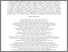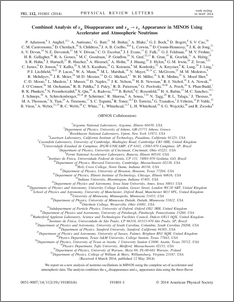# Combined analysis of $ν_μ$ disappearance and $ν_μ \rightarrow ν_{e}$ appearance in MINOS using accelerator and atmospheric neutrinos

, MINOS Collaboration (2014) Combined analysis of $ν_μ$ disappearance and $ν_μ \rightarrow ν_{e}$ appearance in MINOS using accelerator and atmospheric neutrinos. Physical review letters, 112. ISSN 1079-7114Preview
PDF (PhysRevLett.112.191801)
PhysRevLett.112.191801.pdf - Published Version

## Abstract

We report on a new analysis of neutrino oscillations in MINOS using the complete set of accelerator and atmospheric data. The analysis combines the $\nu_{\mu}$ disappearance and $\nu_{e}$ appearance data using the three-flavor formalism. We measure $|\Delta m^{2}_{32}|=[2.28-2.46]\times10^{-3}\mbox{\,eV}^{2}$ (68% C.L.) and $\sin^{2}\theta_{23}=0.35-0.65$ (90% C.L.) in the normal hierarchy, and $|\Delta m^{2}_{32}|=[2.32-2.53]\times10^{-3}\mbox{\,eV}^{2}$ (68% C.L.) and $\sin^{2}\theta_{23}=0.34-0.67$ (90% C.L.) in the inverted hierarchy. The data also constrain $\delta_{CP}$, the $\theta_{23}$ octant degeneracy and the mass hierarchy; we disfavor 36% (11%) of this three-parameter space at 68% (90%) C.L.

Item Type:
Journal Article
Journal or Publication Title:
Physical review letters
Uncontrolled Keywords:
/dk/atira/pure/subjectarea/asjc/3100
Subjects:
Departments:
ID Code:
69934
Deposited By:
Deposited On:
09 Jul 2014 12:04
Refereed?:
Yes
Published?:
Published